# Types Of Vectors

What is a vector?

Vector is a physical quantity that has both direction and magnitude. In other words, the vectors are defined as an object comprising both magnitude and direction. It describes the movement of the object from one point to another. The below figure shows the vector with head, tail, magnitude and direction.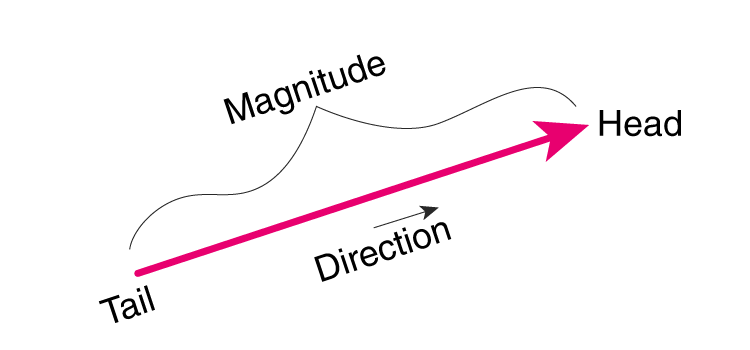There are 10 different types of vectors that are generally used in maths and science. The various vector types that are covered here are as follows.

## Types of Vectors List

There are 10 types of vectors in mathematics which are:

All these vectors are extremely important and the concepts are frequently required in mathematics and other higher-level science topics. The detailed explanations on each of these 10 vector types are given below.

### Zero Vector

A zero vector is a vector when the magnitude of the vector is zero and the starting point of the vector coincides with the terminal point.

$$\begin{array}{l}\text{In other words, for a vector }\overrightarrow{AB} \text{ the coordinates of the point A are the same as that of the} \\ \text{point B then the vector is said to be a zero vector and is denoted by 0.}\end{array}$$

This follows that the magnitude of the zero vector is zero and the direction of such a vector is indeterminate.

### Unit Vector

A vector which has a magnitude of unit length is called a unit vector.

$$\begin{array}{l}\text{Suppose if }\overrightarrow{x} \text{ is a vector having a magnitude x then the unit vector is denoted by } x̂ \\ \text{ in the direction of the vector } \overrightarrow{x} \text{ and has the magnitude equal to 1.}\end{array}$$
$$\begin{array}{l}\text{Therefore, } \hat{x} = \frac{\overrightarrow{x}}{|x|} \end{array}$$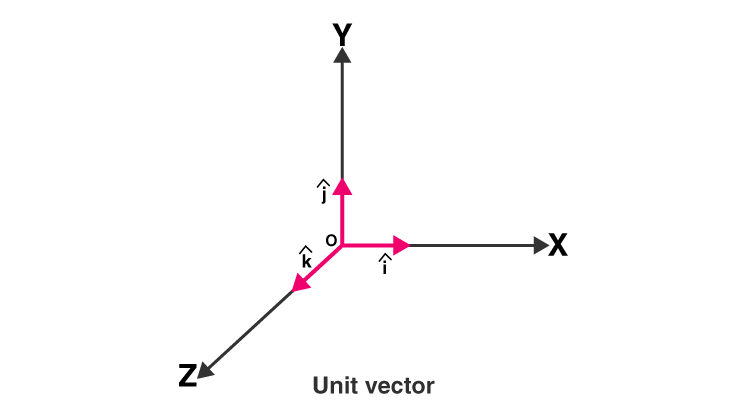It must be carefully noted that any two unit vectors must not be considered as equal, because they might have the same magnitude, but the direction in which the vectors are taken might be different.

### Position Vector

$$\begin{array}{l}\text{If O is taken as reference origin and P is an arbitrary point in space then the vector }\overrightarrow{OP} \\ \text{ is called as the position vector of the point.}\end{array}$$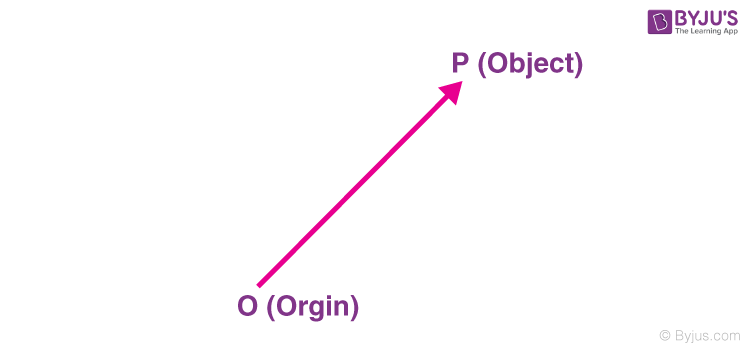Position vector simply denotes the position or location of a point in the three-dimensional Cartesian system with respect to a reference origin.

### Co-initial Vectors

The vectors which have the same starting point are called co-initial vectors.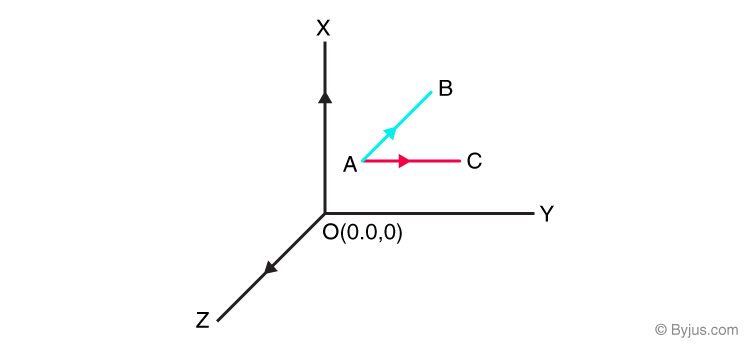$$\begin{array}{l}\text{The vectors } \overrightarrow{AB} \text{ and } \overrightarrow{AC} \text{ are called co-initial vectors as they have the same starting point.}\end{array}$$

### Like and Unlike Vectors

The vectors having the same direction are known as like vectors. On the contrary, the vectors having the opposite direction with respect to each other are termed to be unlike vectors.

### Co-planar Vectors

Three or more vectors lying in the same plane or parallel to the same plane are known as co-planar vectors.

### Collinear Vectors

Vectors that lie along the same line or parallel lines are known to be collinear vectors. They are also known as parallel vectors.

Two vectors are collinear if they are parallel to the same line irrespective of their magnitudes and direction. Thus, we can consider any two vectors as collinear vectors if and only if these two vectors are either along the same line or these vectors are parallel to each other in the same direction or opposite direction. For any two vectors to be parallel to one another, the condition is that one of the vectors should be a scalar multiple of another vector. The below figure shows the collinear vectors in the opposite direction.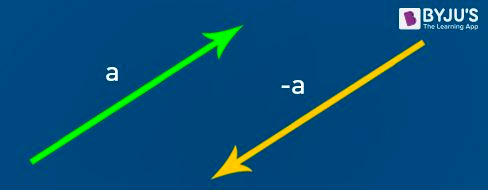### Equal Vectors

Two or more vectors are said to be equal when their magnitude is equal and also their direction is the same.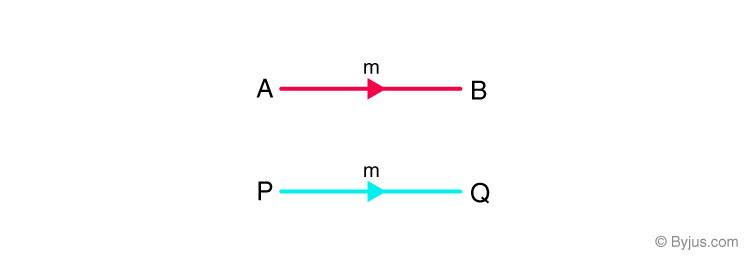The two vectors shown above, are equal vectors as they have both direction and magnitude equal.

### Displacement Vector

$$\begin{array}{l}\text{If a point is displaced from position A to B then the displacement AB represents a vector } \overrightarrow{AB} \\ \text{which is known as the displacement vector.}\end{array}$$

### Negative of a Vector

If two vectors are the same in magnitude but exactly opposite in direction then both the vectors are negative of each other. Assume there are two vectors a and b, such that these vectors are exactly the same in magnitude but opposite in direction then these vectors can be given by

a = – b

These were some basic concepts related to vector types. Check out more vectors related concepts at BYJU’S from the links given below.

Test your Knowledge on Types Of Vectors